GROUP THEORY SYMBOL

# GTSGCosetRepresentative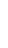GTSGCosetRepresentative[space group, subgroup, basis]
determines a coset representative for a left coset decomposition of the factor group G/T (G being a space group, T the group of pure lattice translations) into a normal sub group of index 2 or 3.

## DetailsDetails

• GTSGCosetRepresentative is mainly needed within the commands GTSGGetIreps and GTSGCharacterTable. Here the underlying algorithm is based on an argument of Zak, stating that every space group contains an invariant sub group of index 2 or 3.
• J. Zak, J. Math. Phys, 1, 165, (1960)
• W. Hergert, M. Geilhufe, Group Theory in Solid State Physics and Photonics. Problem Solving with Mathematica, chapter 6.

## ExamplesExamplesopen allclose all

### Basic Examples  (1)Basic Examples  (1)

•  In:=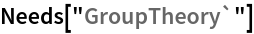• We choose the monoclinic space group P21/c (#14) and one of its subgroups. We first specify the monoclinic basis vectors. Then we install the space groups using GTInstallGroup.
•  In:=Out=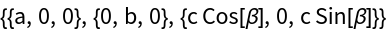In:=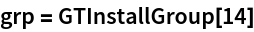Out=In:=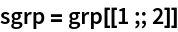Out=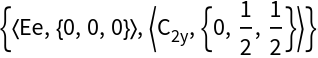• Using GTSGCosetRepresentative we obtain the representative element q as well as a corresponding permutation.
•  In:=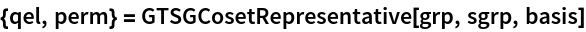Out=• Calculating G = S + q S gives a permutation of the original list G, which can be reconstructed from the calculated permutation.
•  In:=Out=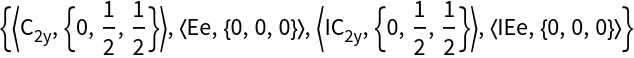In:=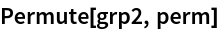Out=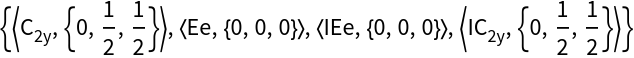In:=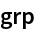Out=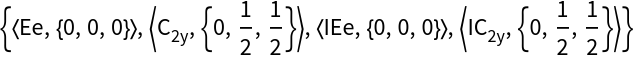GTSGLeftCosetsGTSGRightCosetsGTSGCharacterTableGTSGGetIreps